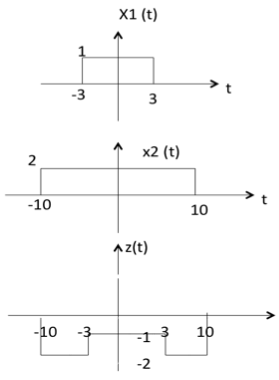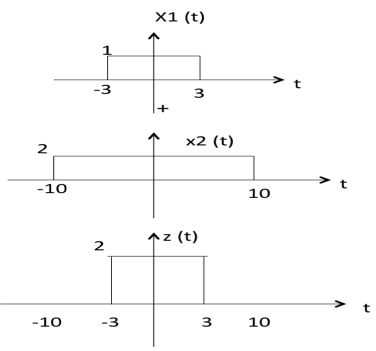# Signals Basic Operations

There are two variable parameters in general:

1. Amplitude
2. Time

The following operation can be performed with amplitude:

### Amplitude Scaling

C x(t) is a amplitude scaled version of x(t) whose amplitude is scaled by a factor C.Addition of two signals is nothing but addition of their corresponding amplitudes. This can be best explained by using the following example:As seen from the diagram above,

-10 < t < -3 amplitude of z(t) = x1(t) + x2(t) = 0 + 2 = 2

-3 < t < 3 amplitude of z(t) = x1(t) + x2(t) = 1 + 2 = 3

3 < t < 10 amplitude of z(t) = x1(t) + x2(t) = 0 + 2 = 2

### Subtraction

subtraction of two signals is nothing but subtraction of their corresponding amplitudes. This can be best explained by the following example:As seen from the diagram above,

-10 < t < -3 amplitude of z (t) = x1(t) - x2(t) = 0 - 2 = -2

-3 < t < 3 amplitude of z (t) = x1(t) - x2(t) = 1 - 2 = -1

3 < t < 10 amplitude of z (t) = x1(t) + x2(t) = 0 - 2 = -2

### Multiplication

Multiplication of two signals is nothing but multiplication of their corresponding amplitudes. This can be best explained by the following example:As seen from the diagram above,

-10 < t < -3 amplitude of z (t) = x1(t) ×x2(t) = 0 ×2 = 0

-3 < t < 3 amplitude of z (t) = x1(t) ×x2(t) = 1 ×2 = 2

3 < t < 10 amplitude of z (t) = x1(t) × x2(t) = 0 × 2 = 0

The following operations can be performed with time:

### Time Shifting

x(t $\pm$ t0) is time shifted version of the signal x(t).

x (t + t0) $\to$ negative shift

x (t - t0) $\to$ positive shift### Time Scaling

x(At) is time scaled version of the signal x(t). where A is always positive.

|A| > 1 $\to$ Compression of the signal

|A| < 1 $\to$ Expansion of the signalNote: u(at) = u(t) time scaling is not applicable for unit step function.

### Time Reversal

x(-t) is the time reversal of the signal x(t).